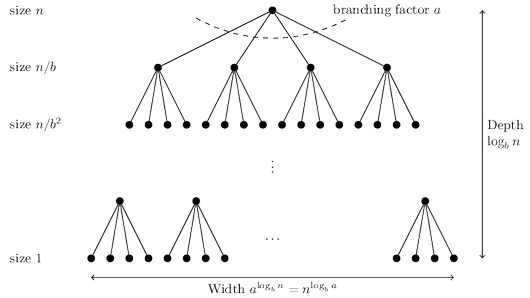# Glossary

## Algo: Master Theorem

Divide-and-conquer algorithms often follow a generic pattern: they tackle a problem of size $n$ by recursively solving, say, $a$ subproblems of size $n/b$ and then combining these answers in $O(n^d)$ time, for some $a, b, d > 0$. Their running time can therefore be captured by the equation $T (n) = aT ( \lceil n/b \rceil) + O(n^d )$. The master theorem gives a closed-form solution to this general recurrence so that we no longer have to solve it explicitly in each new instance.

Master theorem If $T (n) = aT ( \lceil n/b \rceil ) + O(n^d )$ for some constants $a > 0, b > 1$, and $d \ge 0$, $$T(n)= \begin{cases} O(n^d), &\textrm{if } d>\log_ba \, ,\\ O(n^d\log{n}), &\textrm{if } d=\log_ba\, ,\\ O(n^{\log_ba}), &\textrm{if } d<\log_ba\, .\\ \end{cases}$$ Proof. To prove the claim, let’s start by assuming for the sake of convenience that $n$ is a power of $b$. This will not influence the final bound in any important way—after all, $n$ is at most a multiplicative factor of $b$ away from some power of $b$—and it will allow us to ignore the rounding effect in $\lceil n/b \rceil$. Next, notice that the size of the subproblems decreases by a factor of $b$ with each level of recursion, and therefore reaches the base case after $\log_b n$ levels. This is the height of the recursion tree. Its branching factor is $a$, so the $k$th level of the tree is made up of $a^k$ subproblems, each of size $n/b^k$.The total work done at this level is $$a^k \times O\left(\left(\frac{n}{b^k}\right)^d\right)=O(n^d)\times \left(\frac{a}{b^d}\right)^k \, .$$ As $k$ goes from $0$ (the root) to $\log_ b n$ (the leaves), these numbers form a geometric series with ratio $a/b^d$. Finding the sum of such a series in big-O notation is easy, and comes down to three cases.

1. The ratio is less than $1$.
Then the series is decreasing, and its sum is just given by its first term, $O(n^d)$.
2. The ratio is greater than $1$.
The series is increasing and its sum is given by its last term, $O(n \log_b a )$: $$n^d\left(\frac{a}{b^d}\right)^{\log_b{n}}=n^d\left(\frac{a^{\log_bn}}{(b^{\log_bn})^d}\right)=a^{\log_bn}=a^{(\log_an)(\log_ba)}=n^{\log_b{a}} \, .$$
3. The ratio is exactly 1.
4. In this case all $O(\log n)$ terms of the series are equal to $O(n^d)$.
These cases translate directly into the three contingencies in the theorem statement.